# To graph : The quadratic equation y = 2 x 2 − 1000 in a graphing window.### Precalculus: Mathematics for Calcu...

6th Edition
Stewart + 5 others
Publisher: Cengage Learning
ISBN: 9780840068071### Precalculus: Mathematics for Calcu...

6th Edition
Stewart + 5 others
Publisher: Cengage Learning
ISBN: 9780840068071

#### Solutions

Chapter 1.9, Problem 8E
To determine

## To graph: The quadratic equation y=2x2−1000 in a graphing window.

Expert Solution

### Explanation of Solution

Given information:

Graph:

The graph of the quadratic equation y=2x21000 can be sketched in the cartesian plane,

Consider the quadratic equation, y=2x21000 .

Now put the values of x in the equation to find the values of y or to find the real roots of y with the help of x .

Solve the equation y=2x21000 with the quadratic formula:

b±b24ac2a

Now solve the equation y=2x21000 by quadratic formula:

Find the value of a,b,c :

The value of a,b,c are , a is the coefficient of x2 , b is the coefficient of x , c is constant.

Thus, the value of:

a=2b=0c=1000

Substitute the values of a,b,c in quadratic formula:

b±b24ac2a

b±b24ac2a=0±4×2×10002×2=±80004=±10804=±22.36

Either the value ±22.36 is positive or negative:

So x=22.36 or x=22.36 ,

Here observed the equation of parabola, the coefficient of x2 is positive, so the graph is opened upward.

The roots and the shape of parabola is cleared.

The graph of the equation y=2x21000 .

Substitute x=0 , in the equation y=2x21000 ,

y=2x21000y=2(0)21000y=1000

Substitute x=1 , in the equation y=2x21000 ,

y=2x21000y=2(1)21000y=21000y=998

Substitute x=2 , in the equation y=2x21000 ,

y=2x21000y=2(2)21000y=2×41000y=81000y=992

Observe that as the value of x increases there is huge decrement in value of y .

Steps to plot the graph of the equation y=2x21000 with the help of graphing utility are as follows:

Step 1: Press MODE key.

Step 2: Use the down arrow key to reach FUNC option.

Step 3: Press ENTER key.

Step 4: Press Y= key.

Step 5: Enter the function y=2x21000 .

Step 6: Press WINDOW key. Change the settings to

Xmin=100Xmax=100Ymin=1200Ymax=300

For better view of graph.

Step 8: Press GRAPH key.

The result obtained on the screen is provided below,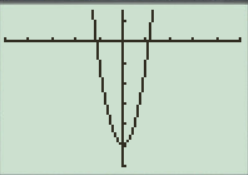Interpretation:

The equation of the function y=2x21000 represents a parabola.

The parabola opens downward.

The x intercepts are the points on xaxis where the graph of the equation touches xaxis .

Therefore, in the equation, y=2x21000x intercepts are [22.5,22.5] .

Therefore, the equation y=2x21000 is symmetric about the y axis Initially the graph of the equation y=2x21000 decreases when increase the value of x ,the graph is a parabola shaped graph.

a.

When the x -axis ranges from [10,10] and y -axis ranges from [10,10] their graph obtained in the screen is provided below.

Steps to plot the graph of the equation y=2x21000 with the help of graphing utility are as follows:

Step 1: Press MODE key.

Step 2: Use the down arrow key to reach FUNC option.

Step 3: Press ENTER key.

Step 4: Press Y= key.

Step 5: Enter the function y=2x21000 .

Step 6: Press WINDOW key. Change the settings to

Xmin=10Xmax=10Ymin=10Ymax=10

For better view of graph.

Step 8: Press GRAPH key.

The result obtained on the screen is provided below,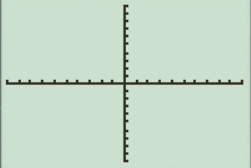This window is not perfect viewing window.

b.

When the x -axis ranges from [10,10] and y -axis ranges from [100,100] their graph obtained in the screen is provided below.

Steps to plot the graph of the equation y=2x21000 with the help of graphing utility are as follows:

Step 1: Press MODE key.

Step 2: Use the down arrow key to reach FUNC option.

Step 3: Press ENTER key.

Step 4: Press Y= key.

Step 5: Enter the function y=2x21000 .

Step 6: Press WINDOW key. Change the settings to

Xmin=10Xmax=10Ymin=100Ymax=100

For better view of graph.

Step 8: Press GRAPH key.

The result obtained on the screen is provided below,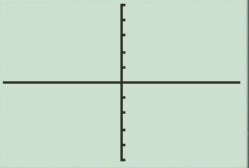This window is not a perfect viewing window.

c.

When the x -axis ranges from [10,10] and y -axis ranges from [1000,1000] their graph obtained in the screen is provided below.

Steps to plot the graph of the equation y=2x21000 with the help of graphing utility are as follows:

Step 1: Press MODE key.

Step 2: Use the down arrow key to reach FUNC option.

Step 3: Press ENTER key.

Step 4: Press Y= key.

Step 5: Enter the function y=2x21000 .

Step 6: Press WINDOW key. Change the settings to

Xmin=10Xmax=10Ymin=1000Ymax=1000

For better view of graph.

Step 8: Press GRAPH key.

The result obtained on the screen is provided below,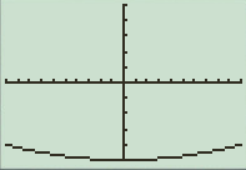This is also the perfect viewing window.

d.

When the x -axis ranges from [25,25] and y -axis ranges from [1200,200] their graph obtained in the screen is provided below.

Steps to plot the graph of the equation y=2x21000 with the help of graphing utility are as follows:

Step 1: Press MODE key.

Step 2: Use the down arrow key to reach FUNC option.

Step 3: Press ENTER key.

Step 4: Press Y= key.

Step 5: Enter the function y=2x21000 .

Step 6: Press WINDOW key. Change the settings to

Xmin=25Xmax=25Ymin=1200Ymax=200

For better view of graph.

Step 8: Press GRAPH key.

The result obtained on the screen is provided below,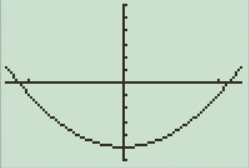This window has perfect view.

So perfect viewing window of the graph y=2x21000 is option ‘ d[25,25]by[1200,200] .

Thus, option ‘ d[25,25]by[1200,200] has a perfect viewing window of the equation y=2x21000 .

### Have a homework question?

Subscribe to bartleby learn! Ask subject matter experts 30 homework questions each month. Plus, you’ll have access to millions of step-by-step textbook answers!